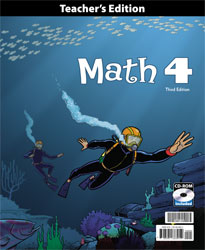US - BJU Math 4 Teacher's Edition with CD (3rd ed.)

• ₱4,180.00

Math 4 (3rd edition) uses manipulatives to build on the mathematical foundations of multiplication, division, and place value, and to develop the student understanding of geometry, fractions, decimals, and measurements. Students read and interpret a variety of charts and graphs. The practical use of the Problem-Solving Plan is emphasized as well as the memorization of addition, subtraction, multiplication, and division facts.

• Edition - 3rd ed.
• Item Code - 260109

We Also Recommend